# Venn Diagram Symbols

Here we will learn about Venn diagram symbols, including common notation, terminology and what the symbols represent.

There are also Venn diagram worksheets based on Edexcel, AQA and OCR exam questions, along with further guidance on where to go next if you’re still stuck.

## What are Venn diagram symbols?

Venn diagram symbols are a collection of mathematical symbols that are used within set theory. Venn diagrams were created by mathematician John Venn and are used widely today within computer sciences, problem-solving and set theory.

To describe a mathematical set using symbols, we need to know the symbols, and what they represent.

Imagine the set of numbers from 1 to 10 .

We can write out the whole numbers (integers) such as 1, 2, 3, etc.

We can also write out the decimals such as 1.5, 6.72 or 0.0003.

We could also write the group of odd numbers, even numbers, prime numbers, factors of \bf{6} , rational numbers (fractions), etc.

All these groups are called sets.

When we list items within a set, we use curly brackets \{ \ \} and separate each item in the list with commas ,

E.g.

The universal set, \xi , of integers from 1 to 10 is written as \{1, 2, 3, 4, 5, 6, 7, 8, 9, 10\} . The set contains 10 items.

If we wrote a subset of this set, for example the even numbers, we would have the set \{2, 4, 6, 8, 10\} . This set contains 5 items.

Note: it is logical to write all the numbers within a set in numerical order, but it is not essential to do this.

The absolute complement, ’ , to the subset of even numbers from 1 to 10 would be the following set \{1, 3, 5, 7, 9\} . This set is the odd numbers from 1 to 10 . The complement of a set is all the items that are not within the subset, but are contained within the larger set.

Finally, we have the empty set, \emptyset , which contains no items. Continuing with the example of the integers from 1 to 10 , an empty subset would be the integers that are odd and even as no item would exist in this set.

So far, the symbols that you should remember are:

You should also be familiar with the sets of natural numbers, integers, real numbers, rational and irrational numbers.

Step-by-step guide: Rational numbers

### What are Venn diagram symbols?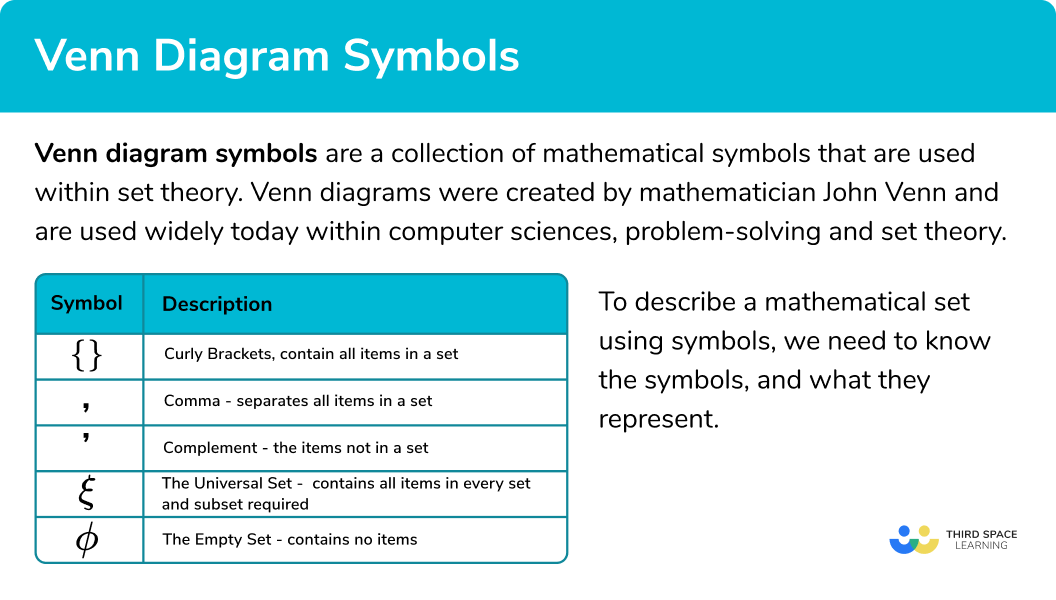### Set notation and shaded regions

Now that we know about what sets look like when we list items within a set, we now need to visualise what different symbols represent when we are looking at sets within a Venn diagram.

We will mainly look at two sets: set A and set B. The shaded region shows the items within the set.

Firstly we start with the universal set, \xi . We represent this as a rectangle and draw the symbol around the outside.

Within the universal set, we have the two sets: set A and set B drawn using two overlapping circles.

The universal set would therefore contain every item within all of the regions in the Venn diagram.

For items within set A, we shade the region:

This is because all of the items that are in the left crescent, and the overlapping middle section are within set A.

Items that are not in set A, the complement of A, would be the following shaded region:

For items in set B, we shade the region:

This is because all of the items that are in the right crescent, and the overlapping middle section are within set B.

Items that are not in set B, the complement of B, would be the following shaded region:

The items that are in set A and set B are called the intersection of A and B. This is the shaded region:

The items that are not in set A and set B, the complement to A \cap B is the shaded region:

This set contains all of the items that are not in the intersection of A and B.

The items that are in set A or set B are called the union of A or B. This is the shaded region:

The items that are not in set A or set B, the complement to AUB, is the shaded region:

## Set notation and shaded regions (part 2)

There are many more sets that we haven’t really considered so far, including those items that are just in set A, items that are in set A and not in set B, and so on. Here is an overview of these set notations for the two sets A and B.

Now we can apply this knowledge to three set Venn diagrams. The table below highlights a few key examples that are useful to remember.

## How to describe a subset of a Venn diagram

In order to determine the set notation for part of a Venn diagram:

1. Determine which sets are included in the shaded region.
2. Determine which sets are not included in the shaded region.
3. State the set notation for the shaded region.

### How to describe a subset of a Venn diagram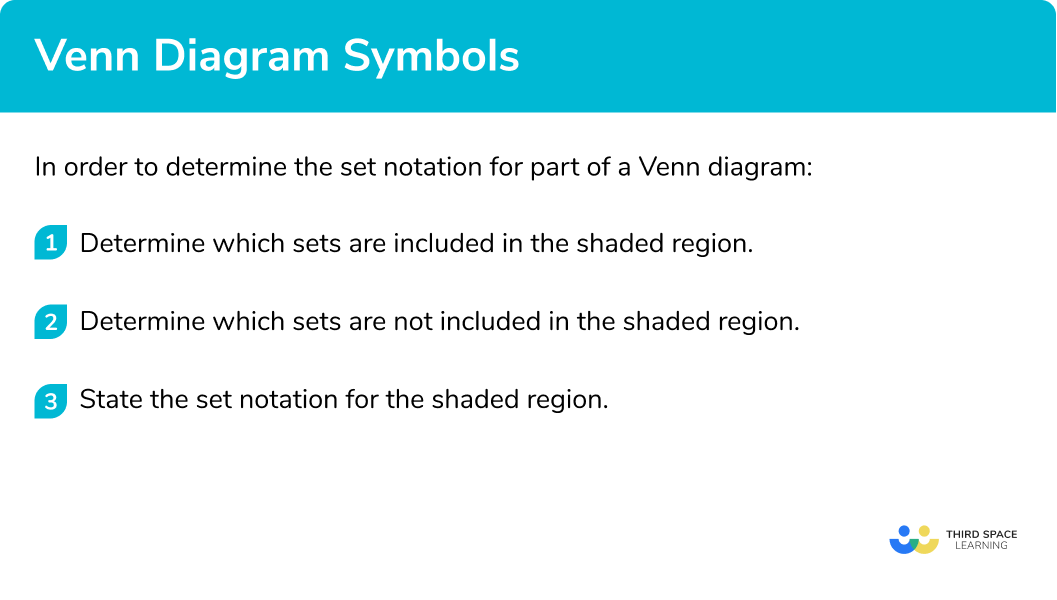## Related lessons on Venn diagram symbols

Venn diagram symbols is part of our series of lessons to support revision on how to calculate probability. You may find it helpful to start with the main Venn diagram lesson for a summary of what to expect, or use the step by step guides below for further detail on individual topics. Other lessons in this series include:

## Venn diagram symbols examples

### Example 1: two set Venn diagram

State the name of the subset that is the shaded region in the Venn diagram below:

1. Determine which sets are included in the shaded region.

Set B and the intersection of set A and set B are included in the shaded region.

2Determine which sets are not included in the shaded region.

Set A only and the outer region are not included in the shaded region.

3State the set notation for the shaded region.

The only set that is shaded is set B.

Solution: B

### Example 2: the intersection of two sets

State the name of the subset that is the shaded region in the Venn diagram below:

The intersection of set A and set B is included in the shaded region.

Set A only, set B only, and the outer region are not included in the shaded region.

The only set that is shaded is the intersection of A and B.

Solution: A \cap B

### Example 3: the union of three sets

State the name of the subset that is the shaded region in the Venn diagram below:

The union of set A, set B and set C is included in the shaded region.

The outer region is not included in the shaded region.

The only set that is shaded is the union of set A, B, and C.

Solution: AUBUC

### Example 4: the complement to a set

State the name of the subset that is the shaded region in the Venn diagram below:

All of set A and the outer region are included in the shaded region

Only items in set B only are not included in the shaded region.

Any item in set A or the complement of set B is included.

Solution: AUB’

### Example 5: the complement to a set

State the name of the subset that is the shaded region in the Venn diagram below:

Items in set A only, set B only and the outer region are included in the shaded region

Items in set A and B are not included.

As the overlapping region is not included, this is the complement to the intersection of A and B.

Solution: (A \cap B)’

### Example 6: a three set Venn diagram

State the name of the subset that is the shaded region in the Venn diagram below:

The intersection of set A and set B without set C is included as well as items in only set B and the outer region.

Items in set A only are not included, as well as all items in set C.

The complement of A union C is included, and the intersection of A and B, without C.

Solution: (AUC)’U(A \cap B \cap C’)

### Common misconceptions

• Using the intersection instead of the union

The two symbols U and \cap have two different meanings. The intersection of two sets means that the item is in both of these sets, whereas the union of two sets, the item can be in one or the other, or both.

• Ignoring items in the universal set only

Remember that the space surrounding the two or more circles can contain items, they simply do not belong to any set, but are part of the universal set.

### Practice Venn diagram symbols questions

1. State the name of the subset that is the shaded region in the Venn diagram below: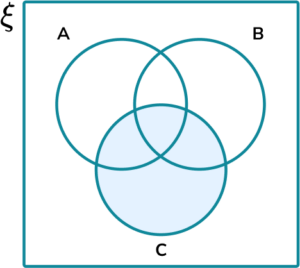CC’(A \cap B)’UCAll of set C is included in the shaded region on the Venn diagram.

2. State the name of the subset that is the shaded region in the Venn diagram below.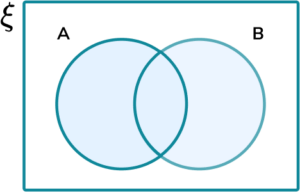A \cap BAUBA’ \cap B’A+BAny item in set A or set B is included. This is the union of A and B.

3. State the name of the subset that is the shaded region in the Venn diagram below: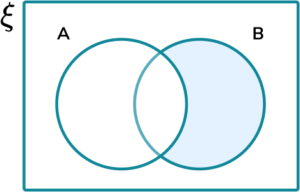A’UBA’B(AUB)’A’ \cap BItems in set B only are included. This does not include items in set B that are also in set A.

4. State the name of the subset that is the shaded region in the Venn diagram below:B’A \cap B(AUB)’AUB’Every item that is not in set A or set B is included. This is therefore not in the union of A or B.

5. State the name of the subset that is the shaded region in the Venn diagram below: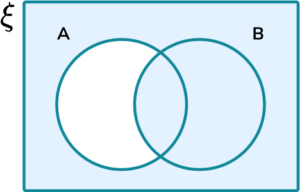A’UB(AUB)’A’UB’B-AThis is the union of two sets: set B and the complement of set A

6. State the name of the subset that is the shaded region in the Venn diagram below: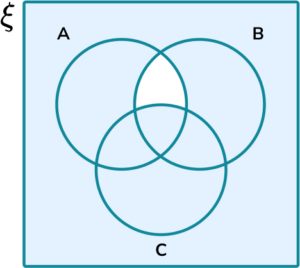(AUC)UB’(AUB)’(AUC) \cap (AUB)’CU(A \cap B)’All of set C is included with the union of the complement of the intersection of A and B.

### Venn diagram symbols GCSE questions

1. The Venn diagram below shows the frequencies of people within the two sets:

• A={Right-handed people}
• B={Male}(a) What does the set A \cap B represent?

(b) What does the set B’ represent?

(c) How many people are in the set AUB?

(3 Marks)

(a) Right-handed people and Male

(1)

(b) Not Male

(1)

(c) 6 + 5 + 3 = 14

(1)

2. The Venn diagram below shows the frequencies of sweets in the three sets:

• A={Fizzy}
• B={Small}
• C={Soft}(a) What does the set AUC represent?

(b) What does the set (BUC)’ represent?

(c) How many sweets are in the set B’?

(3 Marks)

(a) Fizzy or soft

(1)

(b) Not Small or Soft

(1)

(c) 5 + 1 + 4 + 3 = 13

(1)

3. The Venn diagram below shows the distribution of integers within the universal set \xi={x<25} . The two sets A and B represent:

• A={Prime numbers}
• B={Factors of 24 }(a) What does the set A \cap B represent?

(b) What does the set A’UB represent?

(c) How many numbers are in the set AUB’?

(3 Marks)

(a) The prime numbers that are also factors of 24

(1)

(b) Numbers that are not prime or the factors of 24

(1)

(c) 18

(1)

## Learning checklist

You have now learned how to:

• Enumerate sets and unions/intersections of sets systematically, using tables, grids and Venn diagrams

## Still stuck?

Prepare your KS4 students for maths GCSEs success with Third Space Learning. Weekly online one to one GCSE maths revision lessons delivered by expert maths tutors.

Find out more about our GCSE maths tuition programme.